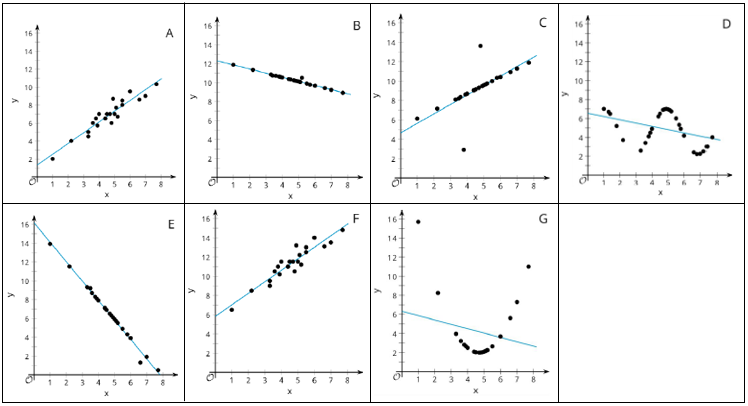# A.3.5.2 Data Patterns

Use the following scatter plots to answer the questions below.Which graph is the best representation of a linear model? Explain why.

Order the linear models from least to greatest slope (leave out graphs that are not a good linear model).

Order the linear models from least to greatest vertical intercept (leave out graphs that are not a good linear model).